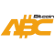Bitcoin ABC  0.22.12 P2P Digital Currency
compressor.h File Reference
`#include <primitives/transaction.h>`
`#include <script/script.h>`
`#include <serialize.h>`
`#include <span.h>`
Include dependency graph for compressor.h:
This graph shows which files directly or indirectly include this file:

Go to the source code of this file.

## Classes

struct  ScriptCompression
Compact serializer for scripts. More...

struct  AmountCompression

struct  TxOutCompression
wrapper for CTxOut that provides a more compact serialization More...

## Functions

bool CompressScript (const CScript &script, std::vector< uint8_t > &out)

unsigned int GetSpecialScriptSize (unsigned int nSize)

bool DecompressScript (CScript &script, unsigned int nSize, const std::vector< uint8_t > &out)

uint64_t CompressAmount (Amount nAmount)
Compress amount. More...

Amount DecompressAmount (uint64_t nAmount)

## ◆ CompressAmount()

 uint64_t CompressAmount ( Amount nAmount )

Compress amount.

Precondition
Function defined only for 0 <= nAmount <= MAX_MONEY.

Definition at line 150 of file compressor.cpp.

Here is the caller graph for this function:

## ◆ CompressScript()

 bool CompressScript ( const CScript & script, std::vector< uint8_t > & out )

Definition at line 53 of file compressor.cpp.

Here is the call graph for this function:
Here is the caller graph for this function:

## ◆ DecompressAmount()

 Amount DecompressAmount ( uint64_t nAmount )

Definition at line 170 of file compressor.cpp.

Here is the call graph for this function:
Here is the caller graph for this function:

## ◆ DecompressScript()

 bool DecompressScript ( CScript & script, unsigned int nSize, const std::vector< uint8_t > & out )

Definition at line 93 of file compressor.cpp.

Here is the call graph for this function:
Here is the caller graph for this function:

## ◆ GetSpecialScriptSize()

 unsigned int GetSpecialScriptSize ( unsigned int nSize )

Definition at line 83 of file compressor.cpp.

Here is the caller graph for this function: